# 基于模糊PID的路径跟踪控制系统Path Tracking Control System Based on Fuzzy PID

• 全文下载: PDF(1009KB)    PP.529-540   DOI: 10.12677/CSA.2020.103055
• 下载量: 28  浏览量: 57   科研立项经费支持

In order to improve the performance of the path tracking control system of the automatic naviga-tion transplanter, a control method based on the fuzzy PID algorithm to adjust the steering angle of the front wheel of the transplanter is proposed. Firstly, the kinematic model of the controlled object is established, and the fuzzy PID control algorithm is designed according to the kinematic model. Secondly, Matlab is used to simulate the path tracking control system. The results show that the performance of the fuzzy PID control method is better than the traditional PID control method, which can effectively reduce the overshoot of the system and the time to reach the steady state. Finally, the dynamic test is carried out to verify that under the traditional PID control method, the maximum path tracking error is 3.8 and 6.5 cm, and the average path tracking error is 2.47 and 3.67 cm when the car chassis is running at the speed of 0.35 and 0.85 m/s; under the fuzzy PID control method, the maximum path tracking error is 2.1 and 4.8cm, and the average path tracking error is 1.57 and 2.7 cm when the car chassis is running at the speed of 0.35 and 0.85m/s. The experiment shows that the performance of fuzzy PID control is better than that of traditional PID algorithm, and it is more suitable for path tracking control of transplanter.

1. 引言

2. 控制系统总体结构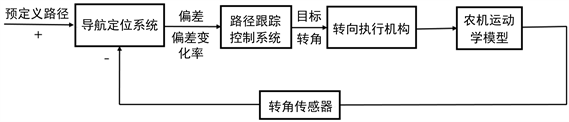Figure 1. Path tracking control principle

3. 运动学模型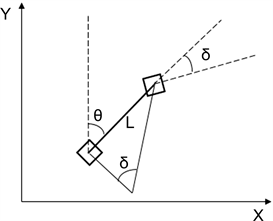Figure 2. Kinematic model of transplanter

$\left\{\begin{array}{l}{x}^{\prime }\left(t\right)=v\mathrm{sin}\theta \\ {y}^{\prime }\left(t\right)=v\mathrm{cos}\theta \\ {\theta }^{\prime }\left(t\right)=\frac{v\mathrm{tan}\delta }{L}\end{array}$ (1)

v：插秧机速度；

$\theta$ ：航向角，取逆时针方向为正；

$\delta$ ：前轮转角，取逆时针方向为正。

4. 模糊PID控制系统

4.1. PID控制

PID控制采用比例、积分和微分的方式对系统进行控制。PID控制分为模拟PID控制和数字PID控制两种方式，本研究用离散化的方法对输入信号进行处理，采用数字PID控制方式来实现对系统的控制 。传统PID控制表示方法：

$u\left(t\right)={K}_{p}\left[e\left(t\right)+\frac{1}{{T}_{i}}{\int }_{0}^{t}e\left(t\right)\text{d}t+{T}_{d}\frac{\text{d}e\left(t\right)}{\text{d}t}\right]$ (2)

${K}_{p}$ ：比例系数；

$e\left(t\right)$ ：输入信号；

${T}_{i}$ ：积分时间；

${T}_{d}$ ：微分时间。

$\Delta u\left(k\right)={k}_{p}\left(e\left(k\right)-e\left(k-1\right)\right)+{k}_{i}e\left(k\right)+{k}_{d}\left(e\left(k\right)-2e\left(k-1\right)+e\left(k-2\right)\right)$ (3)

$e\left(k\right)$ ：输入偏差；

$e\left(k-1\right)$ ：上一次输入偏差；

$e\left(k-2\right)$ ：上两次输入偏差。

4.2. PID控制的参数整定Table 1. Adjustment rules of critical scale method parameters

4.3. 模糊PID控制设计

${K}_{p}={K}_{p1}+\Delta {K}_{p}$ (4)

${K}_{i}={K}_{i1}+\Delta {K}_{i}$ (5)

${K}_{d}={K}_{d1}+\Delta {K}_{d}$ (6)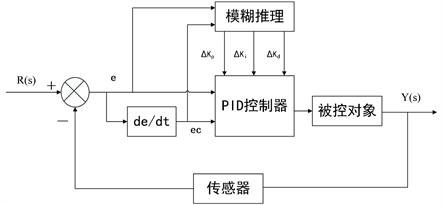Figure 3. Structure of fuzzy PID system

1) 输入、输出变量的模糊化

a) 横向偏差e

b) 偏差变化率ec

c) 比例系数∆Kp

d) 积分系数∆Ki

e) 微分系数∆Kd

2) 定义输入和输出隶属度函数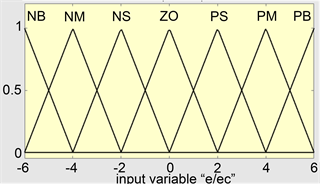Figure 4. Membership function of input variables e and ec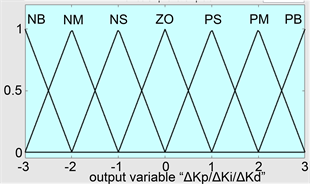Figure 5. Membership function of ∆Kp, ∆Ki, ∆Kd output variables

3) 建立模糊控制规则

a) 横向偏差e和偏差变化率ec的正负表示方向，当横向偏差e的绝对值较大时，系统应快速减小横向偏差e，为提高系统的反应速率，应设置较大的Kp值；提高系统的反应速率会产生微分溢出，为防止微分溢出，应设置较小的Kd值；同时为防止出现积分饱和现象，应设置Ki的值为0。

b) 当横向偏差e的绝对值较小时，为保证系统稳定性良好，Kp值和Ki值应取较大的值；同时为增强路径跟踪控制系统的抗干扰能力，应该选取适当的Kd值，Kd值的具体调整原则：当偏差变化率ec的绝对值较小时，Kd值应取较大值，当偏差变化率ec的绝对值较大时，Kd值应取较小值。Table 2. Kp fuzzy control rulesTable 3. Ki fuzzy control rulesTable 4. Kd fuzzy control rulesFigure 6. ∆Kp output surface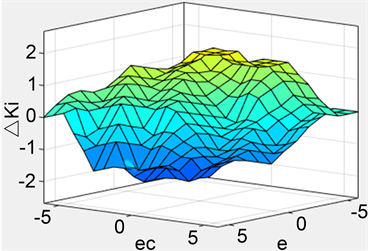Figure 7. ∆Ki output surface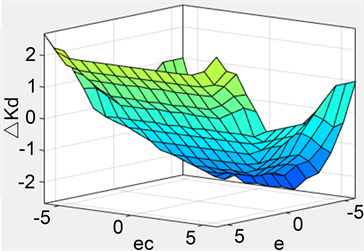Figure 8. ∆Kd output surface

4) 模糊推理和反模糊化Table 5. Fuzzy logic reasoning parameter setting

$u=\frac{\int u\ast {\mu }_{N}\left(u\right)\text{d}u}{\int {\mu }_{N}\left(U\right)\text{d}u}$ (7)

$u=\frac{\sum {u}_{i}\ast {\mu }_{N}\left({u}_{i}\right)}{\sum {\mu }_{N}\left({u}_{i}\right)}$ (8)

5. 系统仿真与试验验证

5.1. 仿真分析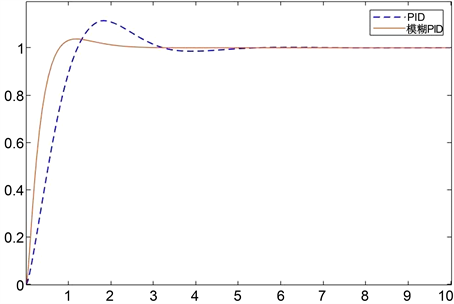Figure 9. Simulation response curve of control system

5.2. 动态试验验证Table 6. Main parameters of trolley chassis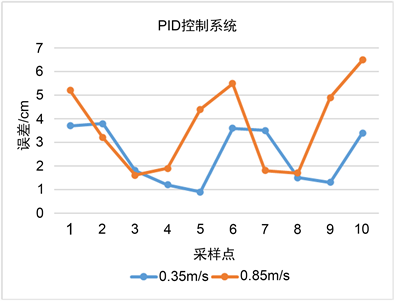Figure 10. Error statistics under PID control method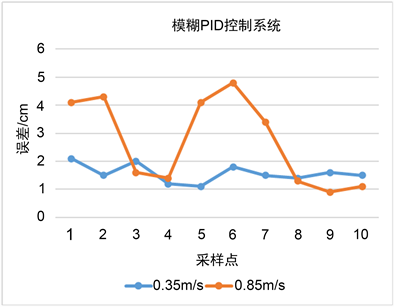Figure 11. Error statistics under Fuzzy PID control method

6. 结论

1) 提出一种基于模糊PID控制算法的自动导航插秧机路径跟踪控制方法。该模糊PID控制相比于传统PID控制算法可减小调整超调量，减少响应时间，可提高系统动态性能。

2) 通过试验验证表明，在模糊PID控制方法下，当小车底盘以0.35和0.85 m/s的速度行驶时，最大路径跟踪误差为2.1和4.8 cm，平均路径跟踪误差为1.57和2.7 cm。验证了模糊PID控制方法性能更优，且对低速和高速都具有适应性。

  谭晨佼, 李轶林, 王东飞, 毛文菊, 杨福增. 农业机械自动导航技术研究进展[J]. 农机化研究, 2020, 42(5): 7-14+32.  迟德霞, 任文涛, 由佳翰, 王洋, 李萍. 水稻插秧机导航控制器设计与路径追踪仿真研究[J]. 沈阳农业大学学报, 2016, 47(3): 363-367.  Wallace, R., Stentz, A., Thorpe, C.E., et al. (1985) First Results in Robot Road-Following. Proceedings of 9th IJCAI, Los Angeles, 18-23 August 1985, 1089-1095.  Amidi, O. and Thorpe, C.E. (1991) Integrated Mobile Robot Control. In: Fibers 91, International Society for Optics and Photonics, Boston, 504-523. https://doi.org/10.1117/12.25494  黄沛琛, 罗锡文, 张智刚. 改进纯追踪模型的农业机械地头转向控制方法[J]. 计算机工程与应用, 2010, 46(21): 216-219.  王辉, 王桂民, 罗锡文, 张智刚, 高阳, 何杰, 岳斌斌. 基于预瞄追踪模型的农机导航路径跟踪控制方法[J]. 农业工程学报, 2019, 35(4): 11-19.  刘正铎, 张万枝, 吕钊钦, 郑文秀, 穆桂脂, 程向勋. 扰动下农用运输车辆路径跟踪控制器设计与试验[J]. 农业机械学报, 2018, 49(12): 378-386.  邹凯. 基于增量线性模型预测控制的无人车轨迹跟踪方法[C]//中国汽车工程学会. 2019中国汽车工程学会年会论文集(1), 北京: 中国汽车工程学会, 2019: 115.  周建军, 张漫, 汪懋华, 等. 基于模糊控制的农用车辆路线跟踪[J]. 农业机械学报, 2009, 40(4): 151-156.  Richa Sharma, K.K., Deepak, P.G. and Deepak, J. (2020) An Optimal Interval Type-2 Fuzzy Logic Control Based Closed-Loop Drug Ad-ministration to Regulate the Mean Arterial Blood Pressure. Computer Methods and Programs in Biomedicine, 185, Article ID: 105167. https://doi.org/10.1016/j.cmpb.2019.105167  罗锡文, 张智刚, 赵祚喜, 陈斌, 胡炼, 吴晓鹏. 东方红X-804拖拉机的DGPS自动导航控制系统[J]. 农业工程学报, 2009, 25(11): 139-145.  Bogler, A., Kastl, A., Spinnler, M., Sattelmayer, T., Beer, A. and Bar-Zeev, E. (2020) Particle Counting and Tracking: Zooming on Deposition and Flow Paths during Initial Stages of Cake Formation in Forward Osmosis with Spacers. Journal of Membrane Science, 597, Article ID: 117619. https://doi.org/10.1016/j.memsci.2019.117619  张曾科. 模糊数学在自动化技术中的应用[M]. 北京: 清华大学出版社, 1997.  童后权. 直流无刷电机中数字式PID控制算法的应用分析[J]. 数字技术与应用, 2014(9): 123.  Bouakkaz, M.S., Boukadoum, A., Boudebbouz, O., Fergani, N., Boutasseta, N., Attoui, I., Bouraiou, A. and Necaibia, A. (2020) Dynamic Performance Evaluation and Improvement of PV Energy Generation Systems Using Moth Flame Optimization with Combined Fractional Order PID and Sliding Mode Controller. Solar Energy, 199, 411-424. https://doi.org/10.1016/j.solener.2020.02.055